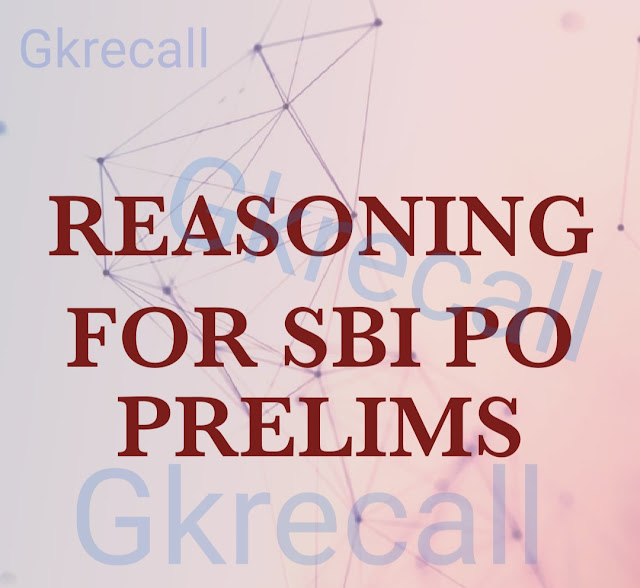# gkrecall

We brings you daily Current affairs, daily Current Affairs Quiz, weekly Current Affairs, weekly Current Affairs Quiz to enhance your preparation for upcoming exams. We also provide articles related to quant, reasoning, English, ssc etc .

## BreakingWe bring you the complete and important daily Reasoning  to achieve more marks in Banking, Insurance, UPSC, SSC, CLAT, Railways and all other competitive Exams. We prepare it based on our daily current affairs.Hope you like it.

START QUIZ
Directions (1 –5):   Study the given information carefully and answer the following questions. Seven persons P, Q, R, S, T, U and V are born in a month of March in different years. Their ages are calculated on the same month with respect to 2018. Note: If it is mentioned that a person’s age is considered as the last two digits of another person’s birth year, then it will be at any sequence. For example, if the age of X is considered as last two digit of birth year of Y which is 1945; then the age of X might be either 45 or 54.
None of the persons were born before 1974 and after 2008.
P was born on1998.The difference between the age of P and T is 5 years. R’s age is equal to the last two digit of birth year of T and his age is not an even number. U’s age is a square number between T’s age and R’s age. S’s age is half of the age of V’s age whose age is last two digit of U’s birth year. The sum of ages of P and S is two more than that of the age of
1. What is the age of S?
A.None of these
B.16 years
C.14 years
D.22 years
E.38 years
2. Which of the following year is the birth year of U?
A.1984
B.1993
C.2000
D. None of these
E.1982
3. If W was born between R and U then which of the following can be the birth year of W?
A.1980
B. None of these
C.1990
D.1982
E.1985
4. What will be the sum of ages of Second youngest and Third eldest person in the group?
A.55 years
B.52 years
C.60 years
D.49 years
E. None of these
5. Which of the following statement is true?
A.U is younger than Q
B.T is three year elder than V
C. None of the options are true
D.S is not the youngest person
E. Number of person born after Q is same as before T
Solution-Direction (6-7): Study the following information carefully and answer the below questions.Among six friends A, B, C, D, E and F each one has different height. C is taller than E. C is shorter than B. B is shorter than both A and F. E is not the shortest. E’s height is 135 cm. The third tallest person is of 165 cm.
6. Who among the following is the tallest?

A. A
B. A or F
C. D
D. C
E. B
7. Who among the following person’s height may be 158 cm?

A. A
B. B
C. D
D. C
E. None of these
Solution-
A/F > F/A > B (165) > C > E (135) > D
Direction (8-10): Study the following information carefully and answer the below questions.There are seven family members A, B, M, N, O, Y and Z and have two married couples. B is the brother in law of Y and son of M. Z is the sister in law of A. M is the grandfather of N. O is the brother of N. M has two children and only one son. Z is the mother of N, who is the niece of A. A is the wife of Y.
8. How is A related to N?

A. Aunt
B. Mother
C. Mother-in-law
D. Sister
E. Sister-in-law
9. If G is the son of A then how is G related to M?

A. Daughter
B. Son
C. Grandson
D. Father
E. None of these
10. How is Z related to O?

A. Mother
B. Father
C. Sister
D. Brother
E. None of these
Solution-